##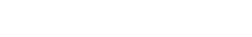Founded By Harris Adams over 40 years ago, Adams-Maxwell Winding Systems has built an industry reputation for quality Bobbin winding and Coil Winding products, and a "customer oriented attitude" dedicated to serving all the needs of our customers.

- Quality andSatisfaction are our Goals

______________________________________________CLICK HERE FOR ORIGINAL ARTICLE IN PDF VERSION________________________________________________

## Power-rating magnetic cores C. Harris Adams, President Coast Magnetics Inglewood, California _________________________________________________________________________

#### Many designers select magnetic cores on the basis of their area product, but this may result in a design that is not optimized. A better approach makes use of a power constant rating that provides a more nearly ideal core size. ______________________________________________________________________________

Over the years, the transformer designer has sought better and faster ways of selecting the proper magnetic structure for a given requirement.  These shortcuts have included rules of thumb, tables compiled from prior design experience, nomographs, model theory and approximations.   Since transformers have been used for several generations, many of these design aids have been in existence for quite some time.  Most have been treated, some exhaustively, in the literature.

Although all types of design short cuts are useful, there appears to have been introduced only two basic methodologies to rate magnetic cores for their power-handling capability. Both are based on model theory. It would be well to note that aids based on model theory are particularly helpful since an extrapolation or modification is easily and quickly made, often as a relatively effortless mental exercise. The ability to easily picture how change in physical structure or operating conditions of a particular design affect the performance is of significant value.

The designer's first challenge as he contemplates a specific requirement is to select the appropriate magnetic structure or core. There is an almost unlimited combination of sizes, shapes and materials from which to choose.  Many of the subtleties of a selection depend heavily on the experience of the designer.  Even so, most magnetic devices are used to either transfer power or to store energy.  For this reason, design criteria usually include volt-amps (VA), which for purposes of this discussion is considered to be synonymous with power. So, this serves as a good place to start the selection process.

What the designer would like to be able to do is rate the various magnetic structures in terms of their ability to handle a specific power (VA).  What appears to be the first and original system to rate for power was developed by Herbert Finch long, long ago.  Mr. Finch demonstrated that cores can be rated in terms of their power-handling capability by a number representing the product of the core area and the core window:

VA · Aw Ac

Where:

Aw is the window area in square inches
Ac is the core area in square inches

This is sometimes referred to as the area product of the core.  The area-product system is quite useful, but the derivation of this relationship is based on the assumption of a specific current density in the winding(s) of the inductor or transformer.  Numerous charts have been published plotting current density against temperature rise for many configurations.  Where temperature rise is the governing design criteria, this is a very satisfactory method of selecting a core.  The constant of proportionality, for example, can be shown to be about 100 for a core with a flux density of 15 kilogauss operating at 60 hertz.  The derivation of this relationship has been reported extensively in the literature and will not be included here.  The primary shortcoming of the area-product rating is that it relates only peripherally to the transformer's regulation and efficiency, which have become increasingly important criteria in recent years.  As a matter of fact, many high-performance cores have such low core loss at low frequencies that the efficiency of the transformer is related primarily to its regulation or copper loss.

If such effects as leakage inductance, skin effect and eddy current losses are ignored, then regulation and efficiency can be considered synonymous.  Put another way, efficiency expressed as a percentage is equal to 100 minus regulation expressed as a percentage.  The efficiency of a transformer designed from regulation criteria in which core loss is significant is still easily calculated by adding the core loss in watts to the regulation loss expressed in watts. Adjusting the design to balance copper loss against core loss is easily done if necessary.

Power Constant
There is, however, another system of rating cores. This system relates the core's power handling capability to a requirement for a specified regulation. The concept has proved of sufficient value to have appeared in manufacturer' catalogs and recent publications under a variety of names. While originally termed the power constant with Kp as the rating symbol, it has more recently been called the regulation factor and the core geometry coefficient .

The formalization of this concept can be handled in a variety of ways, depending upon the designer's individual preference. The basic concept of the Kp rating for cores is based on the following relationships:

VA = Kp ∙ Kc ∙ REG

Where
Kp, the power constant, is a factor related only to core geometry
Kc, the conditions constant, is a factor related only to operating conditions
REG is the regulation the transformer exhibits when delivering a power of a specified volt-amperes

Kp = f (Ac, Aw, Sw, lm)

Where
Ac is the core area
Aw is the window area
Sw is the mean turn length
lm is the copper utilization factor

Kc = f (f, Bm)

Where
f is the frequency of the power being transformed
Bm is flux density within the core
Using these relationships, it can be shown that:
(1)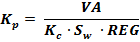Where
(2)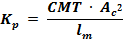And
(3)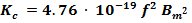In which:
VA = volt-amperes (or watts)
REG = transformer regulation as a percentage
CMT = Total gross circular mil area of the window
Ac = net core area expressed in cm2
Lm = mean winding turn length expressed in feet
f = frequency in hertz
Bm = flux density expressed in gauss.
Sw  = a factor, usually between 0.2 and 0.6, which, when multiplied by CMT, yields the net total circular mils of copper in all windings.

There are three provisos that are well worth noting when applying the power-constant rating method. First, Sw could be included in Kp, but it is of such a variable nature that having external control gives the designer more flexibility. Second, the foregoing is based on proper division of the copper between the windings of the transformer; that is, each winding is allotted copper based on its individual contribution to the total power. Third, the utilization of a mean turn length is an approximation since it is usually different for each winding. However, the second-order error arising from this assumption is normally of small consequence, considering other imprecisions such as the discrete steps between wire gauges. The use of the K-relationship expressed in Equation 1 is straight forward. The value of VA is known. The value of REG is known either from a specific requirement or from an efficiency requirement, or perhaps it is derived from system considerations. The factor Kc is calculated from the operating frequency and the designer's judgment of the flux density, Bm that would logically be used. Finally, the constant Sw, commonly known to be the space factor, is selected. This constant is the ratio of actual copper area that can be placed in the window area to the gross window area. Note that the term "actual copper" means just that and excludes the area filled by insulation, including the insulation on the wire itself.

Note also that Equation 2 is based on the assumption that all windings are loaded for a 100-percent duty cycle, such as that present in a resistive or full-wave bridge load. When windings are used at a 50-percent duty cycle, as they are in full-wave center tapped rectification, the copper utilization in the transformer suffers.  Allowance for this is generally made by modifying the volt-amp requirement.

There is also a proviso for Equation 3: it applies only when the current is in the form of a sine wave. The value 4.76 ∙ 10-19 is valid only for a sine wave.  If other wave forms are pre- sent, a different value must be used to reflect the less effective use of flux changes within the core. It should also be noted that the value shown is valid only when copper conductors are used. Most designers, however, would find it simpler to account for the effects of other wave forms by modifying volt-amp requirements rather than modifying Kc. Some guides for dealing with square wave operation and 50% duty-cycle windings are given in Appendix II.

The value of Kp can now be calculated. Values can be as low as 102 for small cores and as high as 109 for cores commonly used in electronic work.  Large power transformers have Kp values much greater. With the Kp calculation at hand, the designer needs merely to consider core configurations whose Kp rating is at or near the value needed.  Tabulated values of Kp appear in some core manufacturers' catalogs and in some text books, but the values may be labeled differently and may differ from the value calculated by Equation 2. Also, 10 some instances an additional parameter may be introduced. In any event, there is usually one conversion constant that can convert these other values to those given by Equation 2.

The value to the designer of rating cores by their power constant can be better appreciated by considering how its mathematical relationships gives substantial visibility to variations in the design:

1. Regulation varies inversely as CMT
2. Regulation varies inversely as Ac2
3. Regulation varies inversely as f2
4. Regulation varies inversely as Bm2
5. Regulation varies directly as lm

Clearly, the regulation is quite sensitive to the changes in core area, operating frequency and flux density, but is less sensitive to total circular mils and mean turn length. In fitting a transformer in a given space using model theory, the optimum design for lowest regulation almost always involves too much core and too little copper. Using this design generally results in excessive exciting current and/or core loss.

Using a spreadsheet, it is very easy to see all these relationships at one time. With columns for regulation, core loss and efficiency, the optimum design is usually clearly visible.  Besides, the designer is more comfortable with his selection because he knows he has considered a number of alternatives around the chosen design.  A second spreadsheet can be used to calculate turns and wire sizes.

In summary, the designer will find this method of rating cores to be a means of rapid core evaluation and very helpful in the selection of optimum core geometries.  Clearly, other factors can become very important in any particular design.  Higher frequencies, for example, can bring into prominence such factors as leakage inductance and eddy current loss, both of which affect regulation and efficiency.  Nonetheless, the Kr rating of a core will always stand as one of the significant ways of evaluating the core's power-handling capability.

Reference
 C. H. Adams, Power Rating of Magnetic Cores, IEEE Transactions on Magnetics, Vol MAG-6, No 1, Pg 65, March 1970.

APPENDIX I

Model theory is very useful because derivations of complex relationships can often be obtained by making assumptions that only minimally affect the result. Usually, if the required precision of the exercise demands it, first order corrections for the approximations are easily made.
The derivation of Equation 1 assumes that all input power is delivered to the load; that is, the power lost in the winding resistances is neglected. Also, remember that core loss is considered a separate matter. Moreover, a one-to-one ratio transformer is assumed for this discussion. The math to derive the general case is more complex, but the result is the same.

(4)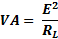(5)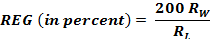Combining Equations 4 and 5:

(6)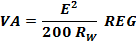From copper resistivity:

(7)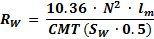From Maxwell's Equation:

(8)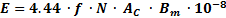Replacing Rw and E in Equation (6) with Equations (7) and (8), and then combining:Referring to Equations (2) and (3), substituting symbology and rearranging: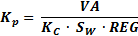The first order correction for the power loss in Rw can be made by using: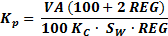APPENDIX II
For square wave operation, Equation 3 would be expressed as: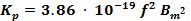Square wave excitation uses the core flux change less efficiently in the transfer of power.  To compensate for this in Equation 1 when dealing with square waves, simply multiply the required VA by 1.23.

Transformers with some or all of its windings operating at a 50-percent duty cycle use the copper in those windings less efficiently.  Because of this, the transformer can deliver less power at a given regulation.  The classic example is the transformer delivering power to a load that includes a half-wave rectifier.  In this case, the regulation would be perhaps three times poorer than it would be at the same power level if the load were a full-wave bridge.

Picture a transformer with a chopper driver primary and a full-wave center tapped secondary load. It is easy to see that only one-half of the transformer is working at a time. The volt-amp capacity in this configuration for the same regulation is one-half that calculated for a 100-percent duty cycle.

A simple approach to use when the transformer operates with 50-percent duty cycle windings is to adjust the power requirement upwards prior to using Equation 1.  The following adjustments can be made:

1. Prior to adding all secondary volt-amp requirements together to obtain the total volt-amp requirement, multiply the volt-amp requirement for each 50-percent duty cycle secondary by 1. 41.
2. If the primary operates with a 50-percent duty cycle, multiply the sum of all secondary volt-amp requirements by 1. 41 after performing Step 1 and use this value in Equation 1.  However, remember that if it is a square-wave application, multiply the final result by 1.23 before using Equation 1.
3.  In the actual design, the division of copper among the windings should be based on the revised volt-amp ratings.